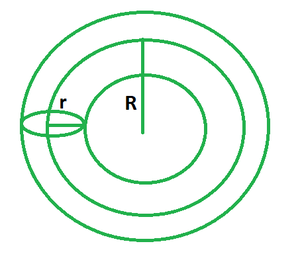Open in App
Not now

# How to find the Volume of a Torus?

• Last Updated : 26 Apr, 2022

In geometry, a torus is defined as a three-dimensional object formed by rotating a tiny circle (with radius r) along a line formed by a larger circle (with radius R). A torus is a surface of the revolution created by rotating a circle in three-dimensional space around an axis that is coplanar with the circle. It has a shape similar to a doughnut. As a result, it has both an inner as well as an outside radius.### Volume of A Torus Formula

A torus is not a polyhedron and has no vertices or edges. If the radius of its circular cross-sectional area is r and the radius of the circle drawn by the centre of the cross-sections is R, the volume of the torus is given by the formula:

V = 2π2r2R

where,

r is the radius of the smaller circle,

R is the radius of larger circle,

π (Pi) is a constant with the value 3.14159.

Derivation

Suppose the torus is obtained by rotating a circular region whose centre is at (0, R) with the equation:

x2 + (y – R)2 = r2

Now, we know this circular region is lying between the curves, x =and y =.

Using Washer method, the volume of the given solid torus can be written as,

V == (4πR) (1/2) (πr2)

= 2π2r2R

This derives the formula for volume of a torus.

### Sample Problems

Problem 1. Find the volume of the torus with an inner radius of 7 cm and an outer radius of 14 cm.

Solution:

We have, r = 7 and R = 14.

Using the formula for volume of torus we have,

V = 2π2r2R

= 2 (22/7)2 (7)2 (14)

= 2 (22) (22) (14)

= 13552 cu. cm

Problem 2. Find the volume of the torus with an inner radius of 14 cm and an outer radius of 18 cm.

Solution:

We have, r = 14 and R = 18.

Using the formula for volume of torus we have,

V = 2π2r2R

= 2 (22/7)2 (14)2 (18)

= 2 (22) (22) (2) (2) (14)

= 54208 cu. cm

Problem 3. Find the inner radius of the torus if its volume is 5040 cu. cm and the outer radius is 14 cm.

Solution:

We have, V = 5040 and R = 14.

Using the formula for volume of torus we have,

V = 2π2r2R

=> 5040 = 2 (22/7)2 (r)2 (14)

=> 5040 = 2 (22/7) (22) (r2) (2)

=> 5040 = 276.57r2

=> r2 = 18.22

=> r = 4.26 cm

Problem 4. Find the outer radius of the torus if its volume is 4400 cu. cm and the inner radius is 7 cm.

Solution:

We have, V = 4400 and r = 7.

Using the formula for volume of torus we have,

V = 2π2r2R

=> 4400 = 2 (22/7)2 (7)2 (R)

=> 4400 = 2 (22) (R)

=> 4400 = 44R

=> R = 4400/44

=> R = 100 cm

Problem 5. Find the difference between the outer and inner radius of the torus if its volume is 2640 cu. cm and the inner radius is 14 cm.

Solution:

We have, V = 2640 and r = 14.

Using the formula for volume of torus we have,

V = 2π2r2R

=> 2640 = 2 (22/7)2 (14)2 (R)

=> 2640 = 2 (22) (4) (R)

=> 2640 = 176R

=> R = 2640/176

=> R = 15

So, the required difference is, R – r = 15 – 7 = 8 cm.

My Personal Notes arrow_drop_up
Related Articles